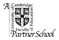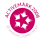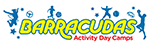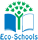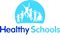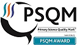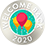# Maths

• Count and calculate in increasingly complex contexts, including those that cannot be experienced first hand.
• Rigorously apply mathematical knowledge across the curriculum, in particular in science, technology and computing.
• Deepen conceptual understanding of mathematics by frequent repetition and extension of key concepts in a range of engaging and purposeful contexts.
• Explore numbers and place value so as to read and understand the value of all numbers.
• Add and subtract using efficient mental and formal written methods.
• Multiply and divide using efficient mental and formal written methods.
• Use the properties of shapes and angles in increasingly complex and practical contexts, including in construction and engineering contexts.
• Describe position, direction and movement in increasingly precise ways.
• Use and apply measures to increasingly complex contexts.
• Gather, organise and interrogate data.
• Understand the practical value of using algebra.